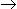# Chemical Engineering - Chemical Reaction Engineering - Discussion

Discussion Forum : Chemical Reaction Engineering - Section 1 (Q.No. 4)
4.
The fractional volume change of the system for the isothermal gas phase reaction, A3B , between no conversion and complete conversion is
0.5
1
2
3
Explanation:
No answer description is available. Let's discuss.
Discussion:
5 comments Page 1 of 1.

Gani said:   8 years ago
This is a gas phase reaction density is not constant.
So e = [Total no of moles of products-Total no of moles of reactants]\Total no of moles of reactants.

Jaydeep jani said:   8 years ago
V = V0(1+(volm at 100% conversion-volm at 0% conversion)/volm at 100% conversion)Xa).

Put Xa=1.
Then,
V = 3V0.

So fractional value changes from 1 to 3.

Sudip chowra said:   8 years ago
Use volume expansion factor.

[(volm at 100% conversion-volm at 0% conversion)/volm at 100% conversion]

Surendra.D said:   10 years ago
The answer is 2: Because one mole of A gives 3 moles of B. And here don't give any conversion fractions. So we are simply use the formula product moles-reactant moles. After divide with reactant moles.

K.srijanya said:   10 years ago
The answer is 2, A---->3B HERE A REACTANT(input) B PRODUCT(output) SO WE HAVE A FORMULA (output-input)/input

By this we can get like (3-1)/1 = 2.